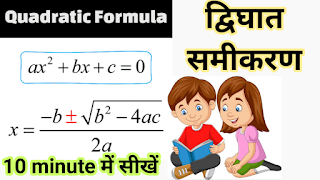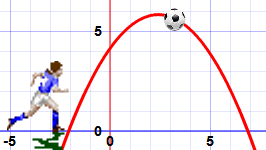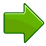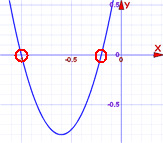## Friday, June 18, 2021

### Trigonometry | Trigonometry hand trick | Trigonometry Class 10 | Trigonometry tricks | त्रिकोणमिति

Trigonometry | Trigonometry hand trick | Trigonometry Class 10 | Trigonometry tricks | त्रिकोणमिति

## Trigonometry | Trigonometry hand trick | Trigonometry Class 10 | Trigonometry tricks | त्रिकोणमिति

Trigonometry | Trigonometry hand trick | Trigonometry Class 10 | Trigonometry tricks | त्रिकोणमिति

## Thursday, June 10, 2021

_________________________________________________

#Youtube Video: Quadratic Formula

An example of a Quadratic Equation:The function makes nice curves like this one:## Name

The name Quadratic comes from "quad" meaning square, because the variable gets  squared (like x2).

It is also called an "Equation of Degree 2" (because of the "2" on the x)

## Standard Form

The Standard Form of a Quadratic Equation looks like this:• ab and c are known values. a can't be 0.
• "x" is the variable or unknown (we don't know it yet).

Here are some examples:

2x2 + 5x + 3 = 0 In this one a=2b=5 and c=3

x2 − 3x = 0 This one is a little more tricky:
• Where is a? Well a=1, as we don't usually write "1x2"
• b = −3
• And where is c? Well c=0, so is not shown.
5x − 3 = 0 Oops! This one is not a quadratic equation: it is missing x2
(in other words a=0, which means it can't be quadratic)

As we saw before, the Standard Form of a Quadratic Equation is

ax2 + bx + c = 0

But sometimes a quadratic equation doesn't look like that!

For example:

In disguiseIn Standard Form a, b and c
x2 = 3x − 1Move all terms to left hand sidex2 − 3x + 1 = 0a=1, b=−3, c=1
2(w2 − 2w) = 5Expand (undo the brackets),
and move 5 to left
2w2 − 4w − 5 = 0 a=2, b=−4, c=−5
z(z−1) = 3Expand, and move 3 to leftz2 − z − 3 = 0a=1, b=−1, c=−3

### Plus/Minus

First of all what is that plus/minus thing that looks like ± ?

The ± means there are TWO answers:

x = −b + √(b− 4ac)2a

x = −b  √(b− 4ac)2a

Here is an example with two answers:But it does not always work out like that!

• Imagine if the curve "just touches" the x-axis.
• Or imagine the curve is so high it doesn't even cross the x-axis!

This is where the "Discriminant" helps us ...

### Discriminant

Do you see b2 − 4ac in the formula above? It is called the Discriminant, because it can "discriminate" between the possible types of answer:

• when b2 − 4ac is positive, we get two Real solutions
• when it is zero we get just ONE real solution (both answers are the same)
• when it is negative we get a pair of Complex solutions

Complex solutions? Let's talk about them after we see how to use the formula.

Just put the values of a, b and c into the Quadratic Formula, and do the calculations.

### Example: Solve 5x2 + 6x + 1 = 0

Coefficients are:a = 5, b = 6, c = 1
Quadratic Formula:x = −b ± √(b− 4ac)2a
Put in a, b and c:x = −6 ± √(6− 4×5×1)2×5
Solve:x = −6 ± √(36 − 20)10
x = −6 ± √(16)10
x = −6 ± 410
x = −0.2 or −1Answer: x = −0.2 or x = −1

And we see them on this graph.

 Check -0.2: 5×(−0.2)2 + 6×(−0.2) + 1= 5×(0.04) + 6×(−0.2) + 1= 0.2 − 1.2 + 1= 0 Check -1: 5×(−1)2 + 6×(−1) + 1= 5×(1) + 6×(−1) + 1= 5 − 6 + 1= 0

### Example: Solve x2 − 4x + 6.25 = 0

Coefficients are:a=1, b=−4, c=6.25
Note that the Discriminant is negative:b2 − 4ac = (−4)2 − 4×1×6.25
= −9
Use the Quadratic Formula:x = −(−4) ± √(−9)2

√(−9) = 3i
(where i is the imaginary number √−1)

So:x = 4 ± 3i2Answer: x = 2 ± 1.5i

The graph does not cross the x-axis. That is why we ended up with complex numbers.BUT an upside-down mirror image of our equation does cross the x-axis at 2 ± 1.5 (note: missing the i).

Just an interesting fact for you!

____________________________________________________________

A quadratic equation is a second-order polynomial equation in a single variable(1)

with. Because it is a second-order polynomial equation, the fundamental theorem of algebra guarantees that it has two solutions. These solutions may be both real, or both complex.

Among his many other talents, Major General Stanley in Gilbert and Sullivan's operetta the Pirates of Penzance impresses the pirates with his knowledge of quadratic equations in "The Major General's Song" as follows: "I am the very model of a modern Major-General, I've information vegetable, animal, and mineral, I know the kings of England, and I quote the fights historical, From Marathon to Waterloo, in order categorical; I'm very well acquainted too with matters mathematical, I understand equations, both the simple and quadratical, About binomial theorem I'm teeming with a lot o' news-- With many cheerful facts about the square of the hypotenuse."

The rootscan be found by completing the square,(2)
 src="https://mathworld.wolfram.com/images/equations/QuadraticEquation/NumberedEquation3.gif" style="border: 0px;" width="217" /> (3)(4)

Solving forthen gives(5)

This equation is known as the quadratic formula.

Here are the steps required to solve a quadratic using the quadratic formula:

## Friday, June 4, 2021

### Adding Polynomials | Polynomials Class 10/9 | Class 10 Maths Chapter 2 | Basics of Polynomial

Adding Polynomials | Polynomials Class 10/9 | Class 10 Maths Chapter 2 | Basics of Polynomial

## Adding Polynomials | Polynomials Class 10/9 | Class 10 Maths Chapter 2 | Basics of Polynomial

Our apps in Play store:

### maths studymaths study 2

__________________________________________________________________________________
_____________________________________________________________________________

Adding Polynomials | Polynomials Class 10/9 | Class 10 Maths Chapter 2 | Basics of Polynomial math,maths,mathematics,polynomials,cbse,class 10,class 10 maths,polynomials class 10,polynomials class 9,class 10 maths chapter 2,class 10 maths chapter,polynomial class 9,polynomial long division,polynomial,polynomial regression,polynomial functions,polynomial equations,polynomial division,minimal polynomial,chromatic polynomial,graph theory,polynomials class 10 cbse,long division,polynomial long division,class 10 polynomials,long division of polynomials,Style stardum MS polynomials full chapter (part 1) class 10,cbse class 10 maths chapter 2,polynomials class 10 cbse,class 10 maths,class 10 maths chapter 2,polynomials class 10 ncert,polynomials class 10,ncert maths class 10,maths class 10 chapter 2,magnet brains,10th maths chapter polynomials,10th maths rs aggarwal,maths class 10 chapter polynomials,class 10 chapter 2 polynomials,10th maths what are polynomials,class 10 maths polynomials introduction,class 10th maths polynomial adding polynomials,Style stardum MS,how to add polynomials,what are like terms?,add like terms,polynomial class 10,polynomial class 9,addition and subtraction,subtract polynomial,column method,additioon and subtraction of polynomials,J.P. PUBLIC ACADEMY,kasganj maths,class 10th chapter 2,chapter 2 polynomial,maths study,math,algebra,polynomials class 10 ncert,class 10 maths chapter 2,polynomials class 10,mathematics,mathematics class 8th,bsda ksj #polynomial #ncert #mathematics #class10th

### Algebraic Expression│Class 7 RS Aggarwal │Introduction of chapter 6

Algebraic Expression│Class 7 RS Aggarwal │Introduction of chapter 6

# Algebraic Expression│Class 7 RS Aggarwal │Introduction of chapter 6│Class 7 chapter 6 in hindi

## What is an Algebraic Expression?

An algebraic expression in mathematics is an expression which is made up of variables and constants, along with algebraic operations (addition, subtraction, etc.). Expressions are made up of terms.  They are also termed algebraic equations.

### Examples

3x + 4y – 7,  4x – 10, etc.

These expressions are represented with the help of unknown variables, constants and coefficients. The combination of these three (as terms) is said to be an expression. It is to be noted that, unlike the algebraic equation, an algebraic expression has no sides or equal to sign. Some of its examples include

• 3x + 2y – 5
• x – 20
• 2x− 3xy + 5

## Variables, Coefficient & Constant

In Algebra we work with Variable, Symbols or Letters whose value is unknown to us.

In the above expression (i.e. 5x – 3),

• x is a variable, whose value is unknown to us which can take any value.
• 5 is known as the coefficient of x, as it is a constant value used with the variable term and is well defined.
• 3 is the constant value term which has a definite value.

The whole expression is known to be the Binomial term, as it has two unlikely terms.

## Types of Algebraic expression

There are 3 main types of algebraic expressions which include:

• Monomial Expression
• Binomial Expression
• Polynomial Expression

### Monomial Expression

An algebraic expression which is having only one term is known as a monomial.

Examples of monomial expression include 3x4, 3xy, 3x, 8y, etc.

### Binomial Expression

A binomial expression is an algebraic expression which is having two terms, which are unlike.

Examples of binomial include 5xy + 8, xyz + x3, etc.

### Polynomial Expression

In general, an expression with more than one terms with non-negative integral exponents of a variable is known as a polynomial.

Examples of polynomial expression include ax by ca,  x3 + 2x + 3, etc.

## Other Types of Expression:

Apart from monomial, binomial and polynomial types of expressions, an algebraic expression can also be classified into two additional types which are:

• Numeric Expression
• Variable Expression

### Numeric Expression

A numeric expression consists of numbers and operations, but never include any variable. Some of the examples of numeric expressions are 10 + 5, 15 ÷ 2, etc.

### Variable Expression

A variable expression is an expression which contains variables along with numbers and operation to define an expression. A few examples of a variable expression include 4x + y, 5ab + 33, etc.

## Algebraic expression for Class 7

In Class 7, students will come across the terms of algebraic equations such as:

• Coefficient of a term
• Variables
• Constant
• Factors of a term
• Terms of equations
• Like and Unlike terms

Example of using these terms are given below.

If 2x2+3xy+4x+7 is an algebraic expression.

Then, 2x2, 3xy, 4x and 7 are the terms

Coefficient of term x2 = 2

Constant term = 7

Example of like and unlike terms:

Like terms: 2x and 3x

Unlike terms: 2x and 3y

Factors of a term:

If 3xy is a term, then its factors are 3, x and y.

Monomial, Binomial & Trinomial

Also, in grade 7 we will learn about types of expressions, such as monomial, binomial and trinomial. Let us see examples of each.

Monomial: 2x

Binomial: 2x+3y

Trinomial: 2x+3y+9

Addition and Subtraction of Algebraic Expressions

We can add and subtract like terms easily.

Example: Add 3x + 5y – 6z and x – 4y + 2z.

By adding both the expressions we get;

(3x + 5y – 6z) + (x – 4y + 2z)

Separating the like terms and adding them together:

(3x + x) + (5y – 4y) + (-6z + 2z)

4x + y – 4z

## Formulas

The general algebraic formulas we use to solve the expressions or equations are:

• (a + b)2 = a2 + 2ab + b2
• (a – b)2 = a2 – 2ab + b2
• a2 – b2 = (a – b)(a + b)
• (a + b)3 = a3 + b3 + 3ab(a + b)
• (a – b)3 = a3 – b3 – 3ab(a – b)
• a3 – b3 = (a – b)(a2 + ab + b2)
• a3 + b3 = (a + b)(a2 – ab + b2)

### Solved Problem

Example: Simplify the given expressions by combining the like terms and write the type of Algebraic expression.

(i) 3xy­­3 + 9x2 y3 + 5y3x

(ii) 7ab2 c2 + 2a3 b2 − 3abc – 5ab2 c2 – 2b2 a3 + 2ab

(iii) 50x3 – 20x + 8x + 21x3 – 3x + 15x – 41x3

Solution:

Creating a table to find the solution:

S.noTermSimplificationType of Expression
13xy­­3 + 9x2 y3 + 5y3x8xy3 + 9x2y3Binomial
27ab2 c2 + 2a3 b2 − 3abc – 5ab2 c2 – 2b2 a3 + 2ab2ab2 c2 − 3abc + 2abTrinomial
350x3 – 20x + 8x + 21x3 – 3x + 15x – 41x330x³Monomial
____________________________________________________________________